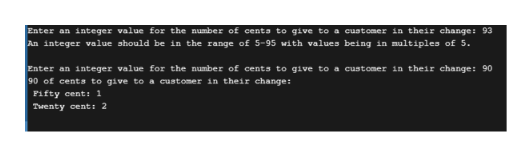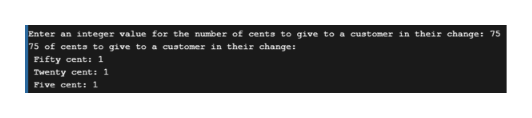# You are asked to write a simple C program that will accept an integer value in the range of 5-95 and in increments of 5 at a time, representing the number of cents to give to a customer in their change. The program should calculate how many coins of each denomination and display this to the user. Valid coin values are 50, 20, 10 and 5. Your solution (program and algorithm) should be modular in nature. This requires the submission of a high-level algorithm and suitable decompositions of each step.

Question
49 views

You are asked to write a simple C program that will accept an integer value in the range of 5-95 and in increments of 5 at a time, representing the number of cents to give to a customer in their change. The program should calculate how many coins of each denomination and display this to the user. Valid coin values are 50, 20, 10 and 5. Your solution (program and algorithm) should be modular in nature. This requires the submission of a high-level algorithm and suitable decompositions of each step.

check_circle

Step 1

Program:

#include <stdio.h>

#include<stdlib.h>

/* Funtion for taking user unput */

int GetInput()

{

int num;

printf("\nEnter an integer value for the number of cents to give to a customer in their change: ");

scanf("%d", &num);

return (num);

}

/* Function to check for negative number */

void negative(int userinput)

{

if(userinput<0)

{

printf("The exit/termination of the program.");

exit(0);

}

}

/* Funtion for varifing user unput */

void checkinput(int *userinput)

{

if ((*userinput < 5) || (*userinput > 95) || (*userinput%5 > 0))

{

printf("An integer value should be in the range of 5-95 with values being in multiples of 5.\n");

*userinput = GetInput();

negative(*userinput);

}

}

/* Function for Calculate coin denominations */

void Change (int *userinput, int *fifty, int *twenty, int *ten, int *five)

{

int number = *userinput;

*fifty = number/50;

number =number%50;

*twenty = number/20;

number =number% 20;

*ten = number/10;

number =number%10;

*five = number/5;

number =number%5;

return;

}

/* Display function */

void Display (int *userinput, int *fifty, int *twenty, int *ten, int *five)

{

printf("%d of cents to give to a customer in their change: \n", *userinput);

if (*fifty > 1)

printf(" Fifty cent: %d\n", *fifty);

if (*fifty == 1)

printf(" Fifty cent: %d\n", *fifty);

if (*twenty > 1)

printf(" Twenty cent: %d\n", *twenty);

if (*twenty == 1)

printf(" Twenty cent: %d\n", *twenty);

if (*ten > 1)

printf(" Ten cent: %d\n", *ten);

if (*ten == 1)

printf(" Ten cent: %d\n", *ten);

if (*five > 1)

printf(" Five cent: %d\n", *five);

if (*five == 1)

printf(" Five cent: %d\n", *five);

}

/* Main function */

int main()

{

//Declared int type varible

int userinput, fifty, twenty, ten, five;

//Calling GetInput()

userinput = GetInput();

//Calling negative()

negative(userinput);

//Calling checkinput()

checkinput(&userinput);

//Calling Change()

Change(&userinput, &fifty, &twenty, &ten, &five);

//Calling display()

Display(&userinput, &fifty, &twenty, &ten, &five);

return(0);

}

Step 2

Output #1:help_outlineImage TranscriptioncloseEnter an integer value for the number of centa to give to a cuatomer in their change: 93 An integer value should be in the range of 5-95 with values being in multiples of 5. Enter an integer value for the number of cents to give to a customer in their change: 90 90 of cents to give to a customer in their change: Fifty cent: 1 Twenty cent: 2 fullscreen
Step 3

Output #2:

...help_outlineImage TranscriptioncloseEnter an integer value for the number of cents to give to a customer in their change: 75 75 of cents to give to a cuatomer in their change: Fifty cent: 1 Twenty cent: 1 Five cent: 1 fullscreen

### Want to see the full answer?

See Solution

#### Want to see this answer and more?

Solutions are written by subject experts who are available 24/7. Questions are typically answered within 1 hour.*

See Solution
*Response times may vary by subject and question.
Tagged in

### Computer Science